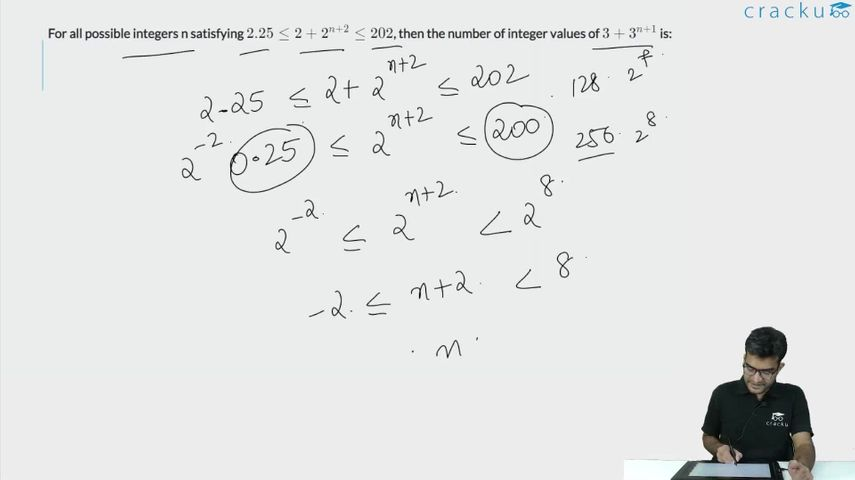Question 45

# For all possible integers n satisfying $$2.25\leq2+2^{n+2}\leq202$$, then the number of integer values of $$3+3^{n+1}$$ is:

Solution

$$2.25\leq2+2^{n+2}\leq202$$

$$2.25-2\le2+2^{n+2}-2\le202-2$$

$$0.25\le2^{n+2}\le200$$

$$\log_20.25\le n+2\le\log_2200$$

$$-2\le n+2\le7.xx$$

$$-4\le n\le7.xx-2$$

$$-4\le n\le5.xx$$

Possible integers = -4, -3, -2, -1, 0, 1, 2, 3, 4, 5

If we see the second expression that is provided, i.e

$$3+3^{n+1}$$, it can be implied that n should be at least -1 for this expression to be an integer.

So, n = -1, 0, 1, 2, 3, 4, 5.

Hence, there are a total of 7 values.

### View Video Solution